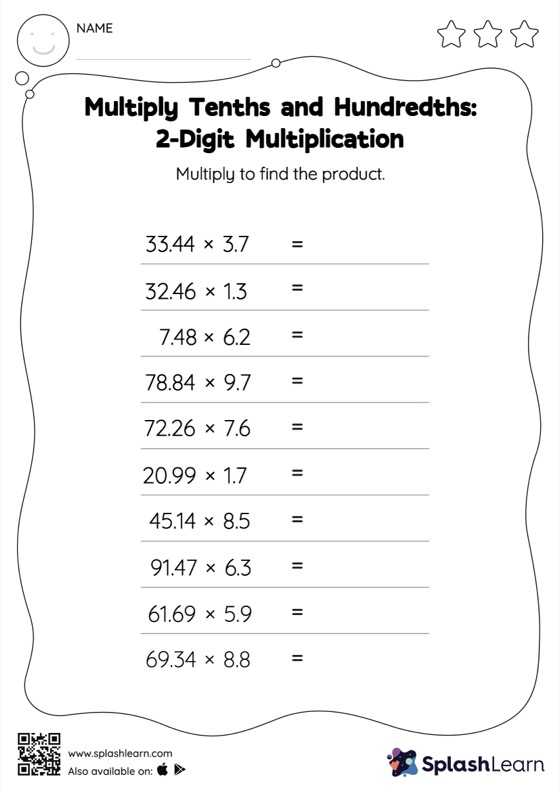# Multiply Tenths and Hundredths: 2-Digit Multiplication: Horizontal Multiplication Worksheet

Home > Multiply Tenths and Hundredths: 2-Digit Multiplication: Horizontal MultiplicationCan your young mathematician multiply tenths and hundredths: 2-digit multiplication? That's exactly what they do here. Students first multiply as though there is no decimal when multiplying two decimals. They then add the same number of digits after the decimal point in the product as there are decimal digits in each number. This multiply tenths and hundredths: 2-digit multiplication worksheet gives students a thorough practice of this concept. In each problem, the numbers are laid out in the horizontal format. Students should try to use different strategies involving composing and decomposing numbers to solve these problems. This will help them develop flexibility and fluency.